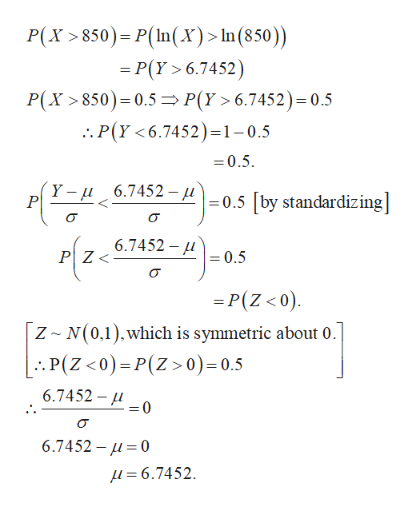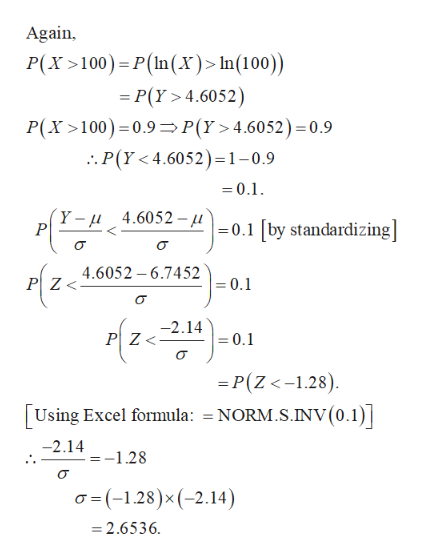The flow in a river can be modeled as a log-normal distribution. From the data, it was estimated that, the probability that the flow exceeds 850 cfs is 50% and the probability that it exceeds 100 cfs is 90%. Let X denote the flow in cfs in the river. What is the standard deviation of log (to the base 10) of X? Please report your answer in 3 decimal places.

Question

The flow in a river can be modeled as a log-normal distribution. From the data, it was estimated that, the probability that the flow exceeds 850 cfs is 50% and the probability that it exceeds 100 cfs is 90%. Let X denote the flow in cfs in the river. What is the standard deviation of log (to the base 10) of X? Please report your answer in 3 decimal places.

Step 1

Introduction:

According to the question, X follows the log-normal distribution. P (X > 850) = 0.5; P (X > 100) = 0.9.

The natural logarithm (base e) of the log-normally distributed random variable with parameters µ and σ, has a normal distribution with mean µ and standard deviation σ. Let Y = ln (X).

Now, it is known that if loga(W) has a normal distribution, then logb(W) also has a normal distribution, as long as a, b > 0. However, the parameter values will change.

Step 2

Calculation:help_outlineImage TranscriptioncloseP(X 850) P(In(x)>In(850) P(Y > 6.7452) P(X 850) 0.5 P(Y>6.7452)= 0.5 . P(Y <6.7452)=1-0.5 =0.5 Y -u 6.7452 - 0.5 by standardiz ing]| 6.7452 PZ 0.5 =P(Z < 0) Z N(0,1),which is symmetric about 0 |.. P(Z <0)=P(Z> 0)= 0.5 6.7452- =0 6.7452- 0 f= 6.7452. fullscreen
Step 3

Calculation continued:...help_outlineImage TranscriptioncloseAgain P(X100 P(In(x)> In(100)) P(Y 4.6052) P(Y>4.6052) = 0.9 P(X100) 0.9 :. P(Y < 4.6052)=1-0.9 0.1 (Y-u4.6052 - 0.1 by standardizing P 4.6052 6.7452 PZ< =0.1 z -2.14 PZ = 0.1 =P(Z<-1.28) Using Excel formula: = NORM.S.INV (0.1) -2.14 -1.28 (-1.28)x (-2.14) =2.6536 fullscreen

Want to see the full answer?

See Solution

Want to see this answer and more?

Our solutions are written by experts, many with advanced degrees, and available 24/7

See Solution
Tagged in

Random Variables